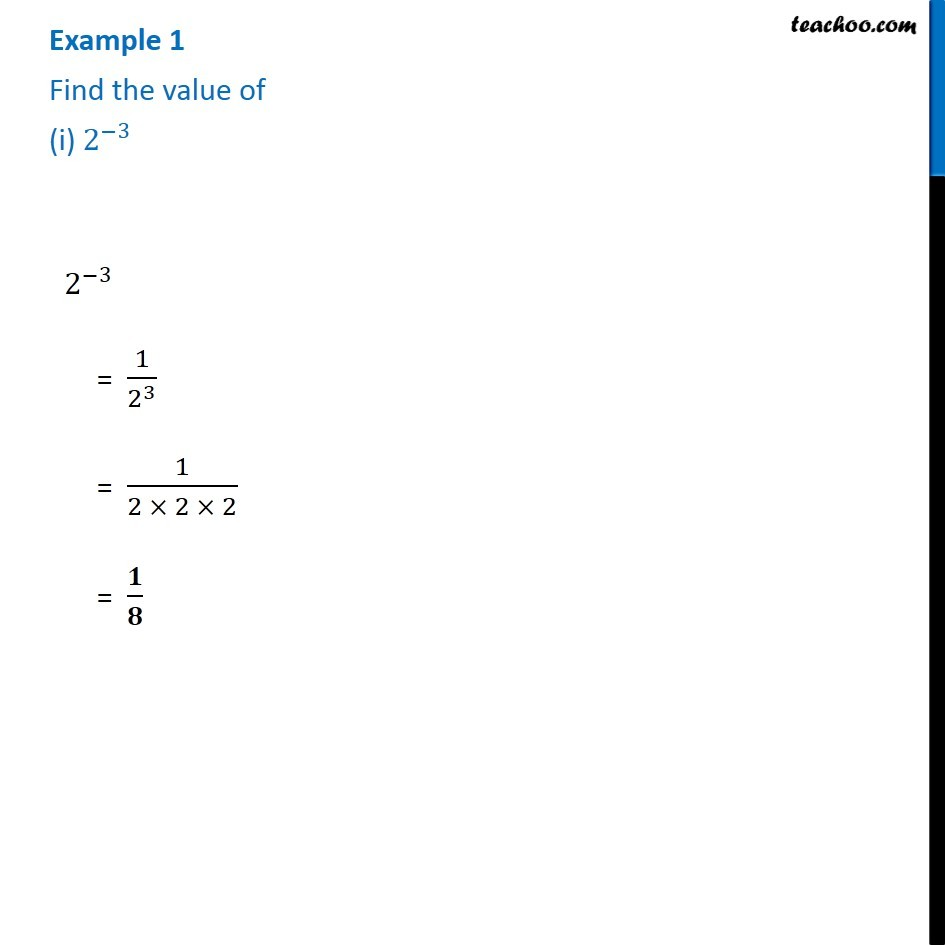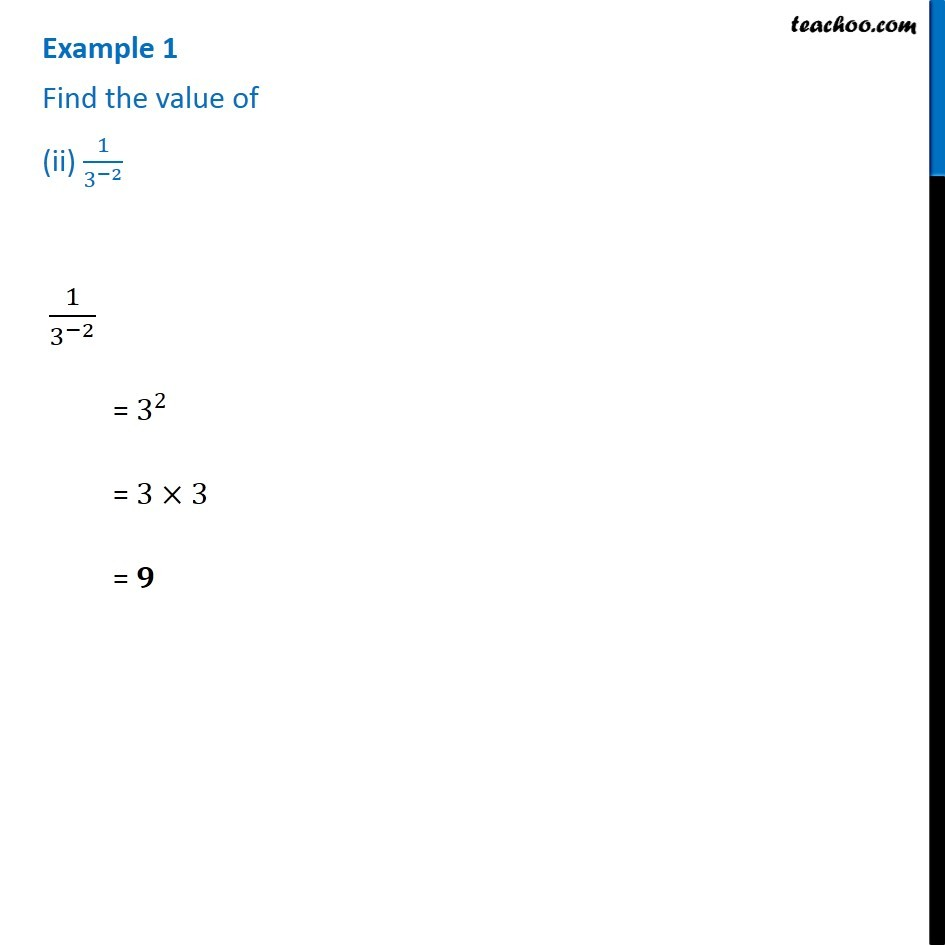1. Chapter 12 Class 8 Exponents and Powers
2. Serial order wise
3. Examples

Transcript

Example 1 Find the value of (i)〖 2〗^(−3) 2^(−3) = 1/2^3 = 1/(2 × 2 × 2) = 𝟏/𝟖 Example 1 Find the value of (ii) 1/3^(−2) 1/3^(−2) = 3^2 = 3×3 = 𝟗

Examples